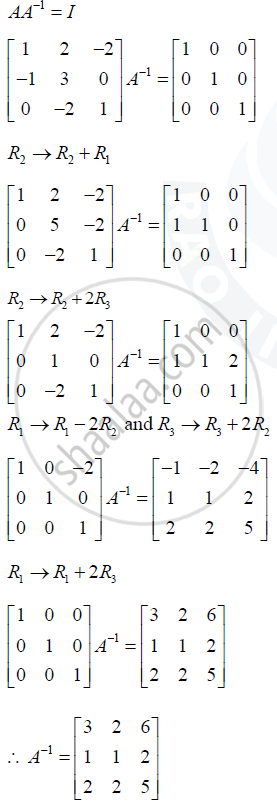# Find the Inverse of the Matrix A = [(1,2,-2),(-1,3,0),(0,-2,1)]Using Elementary Row Transformations. - Mathematics and Statistics

Find the inverse of the matrix A = [(1,2,-2),(-1,3,0),(0,-2,1)]using elementary row transformations.

#### Solution

A = [(1,2,-2),(-1,3,0),(0,-2,1)]

= 1 - 2[-1] - 2

= 3 + 2 - 4 = 1 != 0 => A^(-1) exist

we knowConcept: Matrices - Inverse of a Matrix Existance
Is there an error in this question or solution?